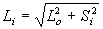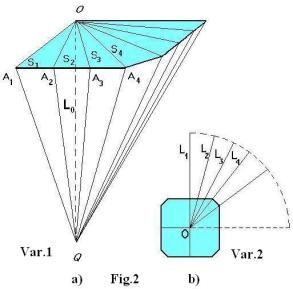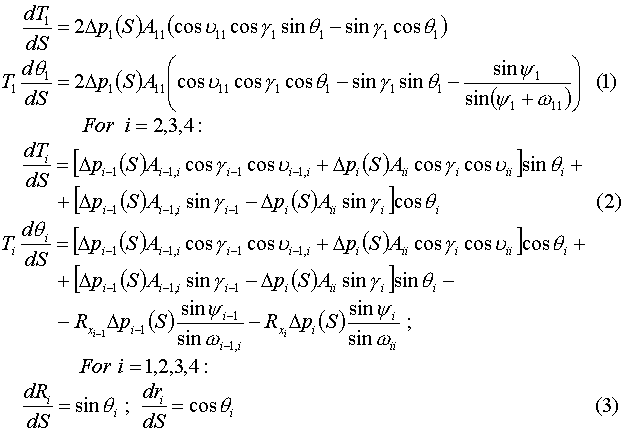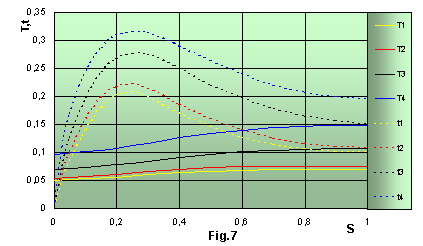### On Square Parachute Differential Equations

#### by Margarita Dzhalalova

 A full system of equilibrium differential equations for the finite number of suspension lines (n = 28) of square parachute is derived. The 8-parameter boundary - value problem for the system of 16 differential equations is solved by means of the developed numerical schemes. One can determine the shapes of inflated canopy radial cross-sections, stress distribution of radial ribbons on the canopy surface and fabric stress between them, drag coefficients for various line lengths and air-permeability by means of computer simulation.

The famous academician Kh.A. Rakhmatulin (he was my Teacher) accomplished the theory of a round parachute . He used on the idea of decisive effect of local radius curvature of a canopy parachute on its shape-formation. For example, scrutinizing the cross-section of an ideal orange, one can see that the edge represents a lot of ideal arcs. By analogue with an orange, the edge of a round parachute consists of a lot of such arcs. The greater the suspension line number, the greater is the arc number. Rakhmatulin's theory allows, first: to determine shape of a round canopy parachute under steady state conditions, taking into consideration all these curvatures between radial ribbons, and second: to determine tension distribution along a canopy. It is enough for deriving the round parachute equations, which describe element's equilibrium of any radial ribbon (thanks to symmetry of all meridional sections relative to the axis).
As for a square parachute, it is more complicated than a round one both theoretically and experimentally. The theory of a square parachute has been developed in papers [2-8].Fig.1: a square parachute in the cutting out with radial ribbon disposition on a canopy with twenty eight lines and cutting off the angles is considered. As one can see the symmetry group of such canopy consists of four sectors, the others are the same. Compared to four differential equations for a round parachute, we need as many as 16 equations to describe the element's equilibrium for a square parachute of a similar construction (this number depends on a symmetry group). If the pressure difference values for a round parachute are constant over total canopy, then for a square parachute the values of pressure difference are non-uniform along radial ribbons differing even from each other.
Two variants of the line length sets are considered. The variant 1 - Fig.2(a): there is 1/4 part of plane square parachute (in the cutting out), where OQ = Lo is the fixed distance from the pole to the point of confluence. Each value of suspension line length for the first variant is defined by Pythagoros formula:i = 1,, because symmetry group consists of four lines. The angle line is the longest in this variant, Si- are lengths of radial ribbons.The variant 2 -Fig.2(b): there is a square parachute in the plane, from the center of which the circumference is drawn with the radius Li + Si . Variant 2 is characterized by the constant sum of line lengths and corresponding radial ribbons for all i. The length of the first line is the same as for variant 1, therefore line lengths with numbers 2, 3 and 4 can be defined by the formula  Li = L1 + S1 - Si , i = 2, 3, 4.
The following Fig.3 presents the scheme of inflated canopy part - one sector. In order to derive the element's equilibrium equations of radial ribbons, each ribbon is divided into the same number of segments (arcs). Cartesian coordinate system is chosen, with the origin on the pole; the canopy axis is directed along ordinate axis.
Each radial ribbon is placed into its plane: for radial ribbon OAoi - this is plane QORi; for OAo,i+1 - plane QORi+1 ; QAoi  and QAo,i+1 - are the two neighbouring lines. The normals AkiNki and Ak,i+1 Nk,i+1  are drawn from the corresponding points Aki and Ak,i+1  to radial ribbons OAoi and OAo,i+1 ; qki is the angle between the axis and the tangent.
The fabric is stretched between neighbouring radial ribbons on the arc of radius Rx . Unlike the round parachute, the normals of square parachute cross with axis OQ in different points Nki , Nk,i+1 . And the distances from points Aki , Ak,i+1  to the axis are not equal to each other, too. The derivation of equations for a square parachute is a sort of boring, therefore the final form of these equations is proposed:All values are given in dimensionless units.  Here index i is the ribbon number. There are 8 equations (1) and (2) of element's equilibrium of the radial ribbons and 8 others (3) determine the shape of radial ribbons of inflated canopy. As the first radial ribbon is perpendicular to canopy edge, then the forces, acting on any elements from left and right, are equal. Therefore these equations are written out separately and have index 1 (1). The equations for the ribbons with number 2, 3, 4 have another form (2). And there are eight equations more (3), analogous to round parachute determining the shape of each of the four ribbons. In all, we have 16 differential equations. This system is complemented by a great number of algebraic relations, determining unknown angles. Unlike the round parachute this system is much more complicated both in solution and boundary conditions. A little about the solution algorithm and boundary conditions of such a system.
So, this system of equations includes 4i differential equations for 4i functions Ti , qi , Ri , and ri .The four boundary conditions 4i are necessary for numerical integration of the constructed system. At the coordinate origin, that is on the canopy pole, where S = 0, one can indicate 3i values of functions: Ri(0) = 0, ri(0) = 0, qi(0) = p/2  (4). The values of tension Ti(0) on the pole are unknown.
The condition Li+ Ri(1)/sinqi(1) = 0,  i = 1, 2, 3, 4  (5)   is realized on the canopy edge (S = 1). Li are the given suspension line lengths. Expression Ri(1)/sinqi(1) has been obtained from the solution of the system. If it is correct, all the expression (5) should be equal to zero.
In all, we have 12 boundary conditions on the pole and 4 on the edge. If we had all the 16 boundary conditions on the pole (that is on one edge), then such problem would be called Cauchy's problem. In our case, we have the part of some boundary conditions on one edge, and the others - on other edge: such a problem is called a boundary-value problem. In spite of the number of formulated conditions formally corresponds to the number of equations, one can not consider this problem as resolved. Initially, this problem was solved assuming Dp = const over total canopy of square parachute.
This four-parameter boundary-value problem has a solution, that is one can find four values of tensions Ti(0) on the pole, for which the boundary conditions are satisfied on the canopy edge. However, it is unexpectedly discovered that line edges do not connect in one point-confluence point. The scatter relative to the distance between pole and confluence point is 8 - 10 per cent. As experimental investigations have shown , the values of pressure difference along the sectors of square parachute for both variants are non-uniform: Dp is a function of dimensionless coordinate S.
Qualitative character of relation p on S is known, but quantitative values of parameters, determining these dependences, are difficult to indicate because of the limitation of experimental data, inaccuracy of measurements, the tremble of the soft parachute models and so on. Nevertheless, one can represent the pressure distributions obtained from experiments, to the first approximation in the form of one-parameter set: Dpi = Dpi(S, Ui) (6), where the parameters Ui can be considered as additional unknowns. So, our system of differential equations is complemented by four algebraic equations (6). The value of parameters Ui should satisfy the conditions, ensuring the connection of all lines in one point-confluence point and parachute equilibrium as a whole. The condition of all line connection in confluence point for the axially symmetric parachutes (like round parachute) is met automatically because the equations of equilibrium are written out only for one element of radial ribbon. This condition in the case of non axially symmetric square parachute corresponds to (i-1) conditions OQ = r1(1) + R1(1)ctgq1(1) = r2(1) + R2(1)ctgq2(1) = r3(1) + R3(1)ctgq3(1) = r4(1) + R4(1)ctgq4(1)   (7)
and the canopy equilibrium as a whole corresponds to one more condition:where n - is the number of lines, P - is the aerodynamic load, acting on the canopy. Thus, the problem of stress state and shapes of square parachute has been reduced to solution of the 8-parameter boundary-value problem for the system of 16 differential equations with boundary conditions (4), (5) for four parameters Ti(0) and additional conditions (7), (8) for four more parameters Ui.
It is impossible to solve such a problem at once, therefore one can offer the next algorithm. As is obvious from the system, the equations of element radial ribbon (number 1) equilibrium contain all terms with index i = 1 (the first ribbon is on an axis of symmetry), so, one can solve these equations separately from the whole system. This procedure has been done for determination of the distance OQ and the tension T1(0). The pressure distribution p along the first section (ribbon) is given in the form of approximating curve, passing through experimental points. This is the first step of problem solution.
It represents the one-parameter boundary-value problem: tension value T1(0) is selected on the pole so that a given value of the first line length would be obtained on the canopy edge. On the pole: R1(0) = 0, r1(0) = 0, q1(0) = p/2; on the edge: L1 + R1(1)/ sinq1(1) = 0   (9)
The second step is the solution of the four-parameter boundary-value problem. The approximating dependences of the pressure distribution for all considered variants are derived as a result of the experimental data processing, i. e. the initial set of parameters Ui is determined. The boundary conditions are the same as such as (9), with index 1 being replaced by index i, which takes the integers 1,2,3,4. In order to make the given values of tensions Ti(0) on the pole closer to the values corresponding to the solution of the full system, one can use the next method.
Using the so-called "shooting method" , we first find the values of Ti(0) on the pole for which the calculated values of line lengths differ from real lines no more then by 30 %. After this procedure, approximating set of values Ti(0) is introduced into another program, where Newton's method is used for the solution of four-parameter boundary-value problem with a necessary precision . (For arbitrary values of Ti(0) solution of this problem would diverge.) After several iterations (their number depends on initial set of parameters Ti(0)), the calculation is finished as soon as boundary conditions are satisfied on the edge to a given precision. The difference between the calculated line lengths and given ones should be no more then 1%. Unfortunately, this is not a final solution. Although the calculated line lengths are obtained to a necessary precision, however, the lines are not connected in one point-confluence point: the scatter relative to the distance OQ is 2 - 4 %. This fact is explained by inaccurate representation of the pressure distribution along all the four radial ribbons.
The last step of calculation is the following: the values of Ti(0) on the pole obtained from the solution of the four-parameter boundary-value problem and initial set of parameters Ui are considered as the first approximation for the solution of 8 - parameter boundary-value problem.The shapes of fourth radial sections for variant 1 with the value of permeability W2 = 600 - 1000 l/m2s and line lengths Lo = 2b are shown in Fig.4. The photos of corresponding parachutes in the stream are placed next to each drawing. The comparison indicates the qualitative correspondence of numerical and experimental shapes of a canopy (the solid curves are results of the system solution). For the quantitative comparison, the shapes of those sections were marked from film by dashed curves, in the plane normal to axes of photo-projection. The connective points of lines on the model edge are shown by asterisks-symbols. Obviously, the section shapes of the first variant parachute differ considerably from the corresponding shapes of the second variant - Fig.5.
The change of line length leads to a change of section shapes:
Ri-coordinates increase and ri-coordinates decrease, that is the profiles of these curves become more slanting. As a consequence, the canopy inlet projection area increases, and, hence, the main aerodynamic characteristic - the drag coefficient - increases too.Drag coefficient Cp via line lengths and permeability W1,2 for var.1 and var.2

In Fig.6 the graphs of the drag coefficient are represented by different experimental symbols according to the upstream flow velocity via the line lengths, permeability W1 and W2 for both variants. Numerical values of the drag coefficient Cp from the system solution are marked by solid curves. One can see that values of Cp agree with the experiments quite satisfactorily.
The lower curve corresponds to variant 2 with W2 . The middle curve corresponds to variant 1 with W2, the upper one - to variant 1 with W1. This variant has the maximum value of Cp. On the abscissa axis there are values of line length Lo = 2b; 3b; 4b; on the ordinate axis there is the drag coefficient. The increase of line lengths from Lo = 2b to Lo = 4b leads to increasing Cp by 30 % for var.2 and by 18 % for var. 1. These results demonstrate the influence of parachute fabric sort (that is permeability W) on the parameters of the shapes and drag coefficient.Distribution of radial ribbon tension Ti and ring-stress t over square canopy

Fig.7- presents the distribution of radial ribbon tension Ti and fabric ring-stress ti between neighbouring meridional sections. Maximum value of t is observed not far from the pole - approximately at S = 0.25 and is several times as much as one on the edge. All these curves have been derived by means of numerical solution. The difference between the values of ring-stress along the first and fourth sectors is 55 - 65 % for var.1.
The distributions of radial ribbon stress T change more smoothly. The values of T on the edge are, approximately, one and half times as many as those on the pole.
Satisfactory agreement of the experimental and numerical values of the drag coefficient Cp and shape parameters of square parachutes at different sets of suspension line lengths and air-permeability indicates that one can reliably predict by the developed calculation scheme both the aforementioned characteristics and parameters of canopy tension - stress distribution of radial ribbon on the canopy surface and fabric stress between them.

##### References
1. Kh.A. Rakhmatulin Theory of Axisymmetric Parachute, Transactions #35, Moscow State University, 1975.
2. M.V. Dzhalalova, Kh.A. Rakhmatulin "Determination of Square Parachute Shape Coefficients". Publication: "Parachute and Permeable Bodies", MSU Publishing, 1980, p.125-131.
3. M.V. Dzhalalova "Theoretical and Experimental Investigations of Shape Coefficients of Square Parachute". Report#3151, MSU, Institute of Mechanics, 1980, p.30.
4. M.V. Dzhalalova "Analysis of Stressed State and Shape-Formation of Square Parachute". MSU Bulletin (Math.& Mech.) 1984, #6, p.93-96.
5. M.V. Dzhalalova "Numerical Investigation of Square Parachute Canopy Tension and Shape in Steady Descent". Report#3189, MSU, Institute of Mechanics, 1985, p.63.
6. M.V. Dzhalalova M.V. "Aerodynamic Characteristics, Shape-Formation and Stressed-Strained State of Parachute Canopy." Dissertation of Ph.D. (Phys.& Math.), 1986, p.241.
7. M.V. Dzhalalova, Kh.A. Rakhmatulin "Investigation of Shape and Stressed State Parameters of Square Parachute Canopy by Numerical Method". Publication: "Parachute and Permeable Bodies", MSU Publishing, 1987, p.63-79.
8. M.V. Dzhalalova, S.V. Guvernuyk, et al "Use of Multichannel Recording Complex for Investigation of Aerodynamic Characteristics of Parachute Systems". Report #4002, 1991, p.49.
9. M.V. Dzhalalova, G.S.Ulianov "Differential Pressure Distribution Peculiarity over Canopy of Square Parachute". Publication: "Parachutes and Permeable Bodies", MSU Publishing, 1987, p.58-62.
10. B.P. Demidovich, I.A. Maron Foundation of Computational Mathematics, Moscow, 1960.
11. N.S. Bakhvalov Numerical Methods, Moscow, 1973.